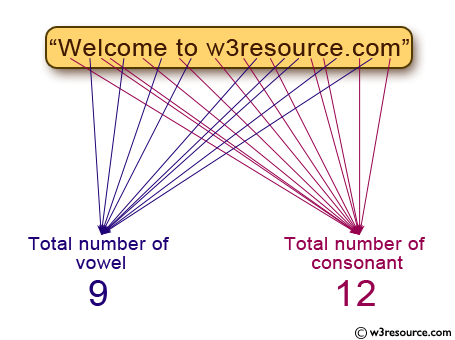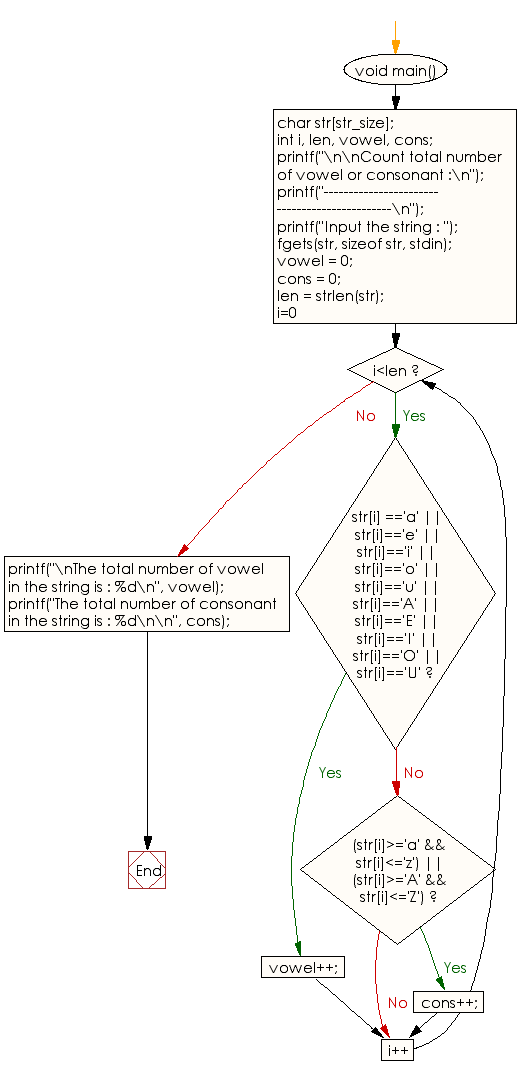﻿ C exercises: Count total number of vowel or consonant - w3resource# C Programming: Count total number of vowel or consonant

## C String: Exercise-9 with Solution

Write a program in C to count total number of vowel or consonant in a string.Sample Solution:

C Code:

``````#include <stdio.h>
#include <string.h>
#include <stdlib.h>

#define str_size 100 //Declare the maximum size of the string

void main()
{
char str[str_size];
int i, len, vowel, cons;

printf("\n\nCount total number of vowel or consonant :\n");
printf("----------------------------------------------\n");
printf("Input the string : ");
fgets(str, sizeof str, stdin);

vowel = 0;
cons = 0;
len = strlen(str);

for(i=0; i<len; i++)
{

if(str[i] =='a' || str[i]=='e' || str[i]=='i' || str[i]=='o' || str[i]=='u' || str[i]=='A' || str[i]=='E' || str[i]=='I' || str[i]=='O' || str[i]=='U')
{
vowel++;
}
else if((str[i]>='a' && str[i]<='z') || (str[i]>='A' && str[i]<='Z'))
{
cons++;
}
}
printf("\nThe total number of vowel in the string is : %d\n", vowel);
printf("The total number of consonant in the string is : %d\n\n", cons);
}
```
```

Sample Output:

```Count total number of vowel or consonant :
----------------------------------------------
Input the string : Welcome to w3resource.com

The total number of vowel in the string is : 9
The total number of consonant in the string is : 12
```

Flowchart:C Programming Code Editor:

Improve this sample solution and post your code through Disqus.

What is the difficulty level of this exercise?

﻿